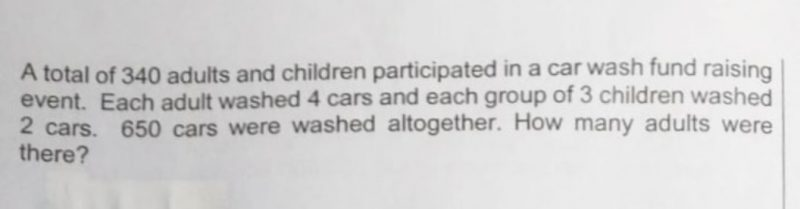# QuestionTeachers pls help.. tia

1 Answer

# Answer

Assume all 340 are adults -> 1360 cars washed

Actual cars washed = 650, hence excess cars = 1360 – 650 = 710

For every instance when 3 adults are replaced by 3 children, fewer cars washed = 12 – 2 = 10

Since there are 710 excess cars, there were 71 such instances -> 71 x 3 = 213 children

Number of adults = 340 – 213 = 127

0 Replies 0 Likes